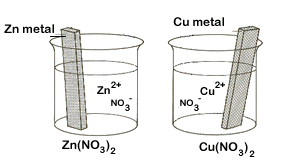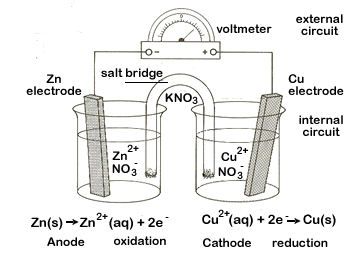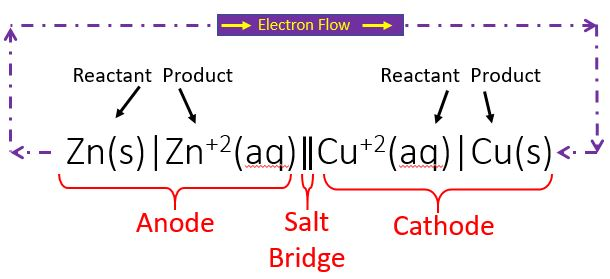# 19.3: Electrochemical Cells

$$\newcommand{\vecs}{\overset { \rightharpoonup} {\mathbf{#1}} }$$ $$\newcommand{\vecd}{\overset{-\!-\!\rightharpoonup}{\vphantom{a}\smash {#1}}}$$$$\newcommand{\id}{\mathrm{id}}$$ $$\newcommand{\Span}{\mathrm{span}}$$ $$\newcommand{\kernel}{\mathrm{null}\,}$$ $$\newcommand{\range}{\mathrm{range}\,}$$ $$\newcommand{\RealPart}{\mathrm{Re}}$$ $$\newcommand{\ImaginaryPart}{\mathrm{Im}}$$ $$\newcommand{\Argument}{\mathrm{Arg}}$$ $$\newcommand{\norm}{\| #1 \|}$$ $$\newcommand{\inner}{\langle #1, #2 \rangle}$$ $$\newcommand{\Span}{\mathrm{span}}$$ $$\newcommand{\id}{\mathrm{id}}$$ $$\newcommand{\Span}{\mathrm{span}}$$ $$\newcommand{\kernel}{\mathrm{null}\,}$$ $$\newcommand{\range}{\mathrm{range}\,}$$ $$\newcommand{\RealPart}{\mathrm{Re}}$$ $$\newcommand{\ImaginaryPart}{\mathrm{Im}}$$ $$\newcommand{\Argument}{\mathrm{Arg}}$$ $$\newcommand{\norm}{\| #1 \|}$$ $$\newcommand{\inner}{\langle #1, #2 \rangle}$$ $$\newcommand{\Span}{\mathrm{span}}$$$$\newcommand{\AA}{\unicode[.8,0]{x212B}}$$

Hypothes.is Tag = c1403c19 (case sensitive)
Group = UALRChem1403

## Introduction

If a redox reaction can be split into half reactions it becomes possible to build a device, called an electrochemical cell, that has separate compartments (cells) for the oxidant and reductant. This physically prevents them from contacting each other and reacting, but allows for charge transfer in the form of electrons through an external circuit and in the form of counter ions in a salt bridge that connects the cells.

In general chemistry 1 we studied a type of reaction called a single displacement reaction (review section 3.2.1.3), where in a metal displacement reaction a pure metal could displace a the cation of an ionic compound. Let's look at the net ionic equations for the two possible single displacement reactions involved with zinc and copper.

$Zn(s) + Cu^{+2} \rightarrow Cu(s) + Zn^{+2} \\ or \\ Cu(s) + Zn^{+2} \rightarrow Zn(s) + Cu^{+2}$

To identify which is spontaneous we used the activity series (review section 3.6.1.1) and from table 3.6a we see that zinc is more active than copper, meaning metallic zinc will spontaneously dissolve in a solution with Copper (II) ions. This means the top reaction above has a negative free energy (it is spontaneous) and could be used to do work, and the bottom reaction above has a positive free energy, which means nothing would happen, unless we added an external source of energy to drive it. In this section we will describe electrochemical cells, and focus on the above reaction to explain how they work. We will show that the spontaneous reactions above ($$Zn(s) + Cu^{+2} \rightarrow Cu(s) + Zn^{+2}$$) can be used to build a battery, which is commonly known as the Daniell cell, that was originally build by the British chemist John Frederic Daniell.Figure $$\PageIndex{1}$$: On the left are two isolated beakers with Zn(s)/Zn+2(aq) and Cu(s)/Cu+2(aq) and nothing happens. On the right is an electrochemical cell which allows electrons to flow from the reductant to the oxidant through an external circuit. In a spontaneous reaction electrons leave the zinc, go through the wire and are then taken up by the copper ions. The salt bridge prevents the copper ions from migrating to the zinc compartment.

Video:$$\PageIndex{1}$$: This 2:54 minute video shows the spontaneous reaction between copper ions and zinc. Note, copper(II)sulfate is a blue solution and the kinetics are speeded up by using fine grained zinc particles (which increases the surface area) and with vigorous stirring it is broken into small pieces to increase the surface area. As the reaction proceeds the blue disappears and a copper colored solid appears on the bottom of the container.

As the above video shows, placing zinc metal into the copper(II) solution results in a spontaneous redox reaction ($$\Delta G <0$$), and if we placed the copper metal into the zinc solution no reaction would occur ($$\Delta G >0$$). In an electrochemical cell, we allow electrons to be transferred, which will spontaneously occur in the direction of $$\Delta G <0$$, and a cell operating in this fashion is called a galvanic or voltaic cell, which can be used to provide energy to do work, like the common "battery" we use in flashlights and electrical devices. Just as we could drive a nonspontaneous reaction by coupling it to a spontaneous reaction (review section 18.6.5), we could hook up an external supply of power with the opposite polarity and drive the reaction in the intrinsically nonspontaneous direction. In this case the electrochemical cell is operating in the electrolytic mode and being driven by an external source of energy.$\underbrace{Zn(s) + Cu^{+2} \rightarrow Cu(s) + Zn^{+2}}_{\text{operating in spontaneous galvanic mode}\\ \; \; \; \;\text{releases energy that can do work}} \nonumber$ $\underbrace{Cu(s) + Zn^{+2} \rightarrow Zn(s) + Cu^{+2}}_{\text{operating in nonspontaneous electrolytic mode}\\ \; \text{ requires external energy to make it work}} \nonumber$

Figure$$\PageIndex{2}$$: The two modes an electrochemical cell operates in. The left mode is the spontaneous redox reaction and can be used to provide energy to do work. The right mode is the electrolytic mode, which will not occur unless an external energy source is added to drive it. That is, the reaction on the electrolytic cell is not spontaneous unless it is driven by an external source

Sign Convention of Galvanic and Electrolytic Cells.  Students are often confused that the sign convention of the anode and cathode switch for galvanic and electrolytic cells. What you need to realize is oxidation occurs at the anode and electrons leave the anode, and reduction occurs at the cathode, this is always true.  In the Galvanic cell is is easy to see, and electrons flow from the Anodes through a load (light bulb) and to the cathode [+].  Now in an electrolytic cell the electrochemical cell sort of becomes like the load on the galvanic cell, and the sign of the electrodes are defined by the power supply. The copper electrode is hooked to the [+] electrode and there is no load between it and the electrode, so it is the [+] electrode, but it is the anode as electrons flow from it to the [+] electrode of the power supply, and then to the [-] anode.  Simply speaking, the electrolytic cell must have an external power supply that drives the reaction, and the sign is based on the electrodes of the power supply

## Components of Electrochemical Cells

An electrochemical cell splits the oxidant and reductant in a manner that allows electrons to flow through an external circuit from the reductant (which gets oxidized) to the oxidant (which causes reduction) while preventing them from physically touching each other. Figures 19.2.1, 19.2.2 and 19.2.3 represent electrochemical cells and you should look at those images while reading this section. The two compartments of an electrochemical cell where the half reactions occur are called the anode and the cathode, and they must have an electrode that you can connect the external circuit to.

• Anode: Oxidation occurs at the anode. This is always the case, but the sign of the anode switches between galvanic and electrolytic modes (see figure 19.2.2).
• In a galvanic cell this is the negative electrode. This can be understood from two perspectives. From the reaction perspective, as the reductant (Zinc in the images on this page) lose electrons and enter the solution the electrode gains these electrons and thus acquires a negative charge, which can be transferred to something positive. The second is from the perspective of the external circuit, where the negative electrons flow to the positive terminal, which is the other electrode, making the anode the negative electrode
• In an electrolytic cell this is the positive electrode. Here the electrode sign is not being determined by the cell reaction, but by the external power supply that is driving the reaction in the nonspontaneous direction. The right side of figure 19.2.2 shows the Daniel Cell being driven by an external power supply which has its [+] electrode connected to the cell with the copper. This causes the nonspontaneous reaction of electrons leaving the copper and moving towards the positive electrode. Since the wire connecting this to the power source has negligible resistance, this electrode is at roughly the same potential, and is thus the positive electrode. It must be emphasized that the reaction is being driven by the coupling of an external power supply that produces a negative free energy in the nonspontaneous direction, and this must be greater in magnitude than the spontaneous free energy that occurs in the galvanic cell, resulting in a net negative free energy that causes the intrinsically nonspontaneous reaction to occur. Because of this, the electrode sign is dictated by the external power supply. But the anode is still where oxidation occurs.
• Cathode: Reduction occurs at the cathode.
• The cathode has the opposite signs of the anode, so it is [+] in a galvanic/voltaic cell, and [-] in an electrolytic cell.
• Electrodes: In order to hook up an external circuit you need have something to physically connect the wire to. There are two types of electrodes, active and inert.
• Active electrode: This is an electrode that takes part of the half reaction, and thus must be a solid. In the Daniels (Zn+2/Cu) cell both electrodes are active, and as the reaction proceeds the anode loses mass (as Zn converts to Zn+2 and enters the solution), while the cathode gains mass (as Cu+2 gains electrons from the external circuit and becomes Cu(s).
• Inert electrode: This is an electrode that is made of a substance that does not undergo oxidation or reduction. Often times oxidants or reductants are not solids but gases or solutes, like in the hydrogen half reaction $$2H^+(aq) +2e^- \leftrightharpoons H_2(g)$$, which has no solid material that can function as an electrode, and would thus require an additional substance like platinum or graphite, that would function as an inert electrode.
• Electrolyte: An electrolyte is a charged mobile ion that functions as a conducting medium and in the case of "wet cells" is typically an aqueous solution of ionic compounds. In the case of active electrodes this will often by a soluble salt that has a common ion that also makes a soluble salt with the metal of that half-cells electrode. For example, copper(II) sulfate is a soluble salt, so sodium sulfate would be a good electrolyte for that half cell. Sodium phosphate would not work because copper(II)phosphate would precipitate out.
• External Circuit: The external circuit allows electrons to flow from the anode to the cathode. In the case of a galvanic cell, this is a spontaneous flow and can be used to provide energy that can produce heat or do work. In the case of an electrolytic cell this requires an energy source to make it work.
• Salt Bridge: The salt bridge has two basic functions. First it completes the circuit and prevents charge buildup, which would stop the flow of electrons. That is, electrons flow towards a positive object and as they accumulate they reduce its "positiveness", and thus its ability to attract more electrons. The salt bridge prevents the buildup of negative charge by enabling an internal circuit that allows ions to complete the circuit and prevent charge buildup at the electrode.

The second function of the salt bridge is to prevent the oxidant (Cu+2 in the Daniel cell) from migrating to the anode and being directly reduced by directly accepting electrons from the anode, and thus preventing the electrons from entering the external circuit. Thus it functions as a sort of "semipermeable membrane" allowing the electrolyte ions to freely migrate between half cells, but preventing the ions involved in the actual redox reaction from migrating. In reality some of the ions involved in the redox reaction do migrate through the salt bridge.

## Cell Notation

Cell Notation is a shorthand way for describing an electrochemical cell. In cell notation you start with the anode on the left, and write down the reactants and products separated by a vertical line, which indicates a phase boundary (the salt bridge involves two phase boundaries). It should be noted that there needs to be a solid electrode at the very left of the anode and very right of the cathode. Finally, it is also important to note that these do not represent balanced equations, but identify what is being oxidized and what is being reduced.Figure$$\PageIndex{3}$$: Cell notation for zinc copper cell operating in galvanic mode. Note, the purple dotted line is not part of the cell notation, but shown here. That is, the above would be written as Zn(s)|Zn+2(aq)||Cu+2(aq)|Cu(s).

If the ion concentrations were known the (aq) would be changed with the molarity. So at standard one molar conditions the zinc copper cell would be written as

$Zn(s)|Zn^{+2}(1M)||Cu^{+2}(1M)|Cu(s)$

If the above cell was operating in electrolytic mode it would be written as

$Cu(s)|Cu^{+2}(aq)||Zn^{+2}(aq)|Zn(s)$

The half cell notation does not represent a balanced equation, and the ends must be solid, as they function as the electrode.

Example $$\PageIndex{1}$$: Cell Notation

What is the cell notation for the redox reaction:

$\ce{Cu(s) + 2Fe^{3+}(aq) \rightarrow Cu^{2+}(aq) + 2Fe^{2+}(aq)}$

Solution

This can be broken into the following oxidation reactions

• Oxidation: $$\ce{Cu(s) \rightarrow Cu^{2+}(aq) + 2}e^{–}$$
• Reduction: $$\ce{Fe^{3+} + }e^– \ce{\rightarrow Fe^{2+}}$$

Which gives the cell of

$\ce{Cu} \mid \ce{Cu^{2+}} \parallel \ce{Fe^{3+}, Fe^{2+}} \mid \ce{Pt} \label{19.2.5}$

There are three things to note in Equation \ref{19.2.5}.

1. First, the reduction half reaction involves two ions and so there is no solid, and an inert electrode had to be added (Pt) to the end of the cathode half reaction.
2. Second, the Fe+2 and Fe+3 are in the same phase and so you use a comma to separate them and not a vertical line (the vertical line represents a phase boundary).
3. And third, you are only identifying what is being oxidized (in the anode) and reduced (in the cathode), and so the cell notation does not represent a balanced chemical equation.

## Voltaic (Galvanic) Cells

Galvanic cells are electrochemical cells that can be used to do work. Figure$$\PageIndex{3}$$ shows a typical galvanic cell that uses the spontaneous (Zn+2/Cu) reaction (eq. 19.2.1 above). If the Zn+2 and Cu+2 ion concentrations in the two half cells is 1M a volt meter will read 1.10 volts. This is actually the open circuit potential that the voltmeter is reading, which correlates to no current flowing. The moment current flows [Zn+2] increases while the [Cu+2] decreases and this can be seen by the images in part (b) of figure 19.2.3 as the Zinc anode dissolves and copper ions attach themselves to the electrode and become reduced to neutral copper.Figure$$\PageIndex{3}$$: Daniell Cell using the spontaneous (Zn+2/Cu) reaction.

Exercise $$\PageIndex{1}$$

Are the electrodes of the (Zn+2/Cu) cell that is described in figure 19.2.3 active or passive electrodes?

Active electrodes because they take place in the Redox rection. This can be seen by the image on the right that shows the zinc anode losses mass as the copper cathode gains mass. In fact, if we measure the mass change of either the anode or cathode over time we could determine the number of electrons transferred, and calculate the average current over that time span that would have resulted in that mass change.

Note

We typically use the word "battery" to mean a galvanic (or voltaic) cell that can be used to power electrical devices, but that is technically incorrect and a battery can be galvanic or electrolytic. The term battery originally came from a series of cannons, and a battery of electrochemical cells represent a series of cells that are linked in series. Your 12 volt car battery is actually six 2 volt cells linked in seriesFigure$$\PageIndex{3}$$: Left is a Union Civil War artillery battery from the national archive, and to the left is an image of a carbon arc lamp being run by a battery of galvanic cells (wikicommons). Arc lamps predated incandescent lamps and were first demonstrated by Sir Humphry Davy in 1806, and by 1807 he had a high voltage battery of 3,000 cells that enabled him to discover sodium and potassium through the electrolysis of soda and potash. The following year he discovered magnesium, strontium and calcium.

Electronic Schematic Symbol of a battery implies cells connected in seriesFigure$$\PageIndex{3}$$: The symbol used in circuit diagrams for a battery. The large vertical line is a cathode and the small is the anode, and this image implies two cells connected in series. In reality there can be many cells. Note, in electronics the convention of flow is the direction positive charge would flow, and this can cause confusion. That is, diagrams would show current flowing through an external circuit from the left electrode to the right electrode, while in reality, electrons are flowing from the right electrode (anode, where oxidation occurs) to the left electrode (cathode, where reduction occurs).

Homework